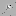## fullrun_ccd6_acisf11016_pi14-548_cl_pass1_csvfiles

#### Source 1

Data taken from csv/fullrun_ccd6_acisf11016_pi14-548_cl_pass1_tfrozen_src1.csv
X043200.40+180055.3
C/dof = 932.79/3014
Total counts = 116
Model total counts = 112.7
1 const = 0.03613 (0.0291 - 0.04377)
Model 1
Model 1
2 linx = 0.0009405 (-0.0002918 - 0.002059)
3 liny = 0.0001946 (-0.0008869 - 0.001271)
Model 2
Model 2
4 xc = -13.51 (indef)
5 yc = 13.58 (indef)
6 norm = 1e-06 (<0.275)
7 sigmax = 1.098 (indef)
8 sigmay = 1.098 (= 7)
9 theta = 0 (frozen)
Model 3
Model 3
Model 3 Position (J2000) = 4 32 0.40 18 0 55.3 (68.001664, 18.015366)
Model 3 Position (xc, yc) = (77.541870, 483.407520)data model resid

#### Source 2

Data taken from csv/fullrun_ccd6_acisf11016_pi14-548_cl_pass1_tfrozen_src2.csv
X043156.00+175913.6
C/dof = 520.43/1707
Total counts = 65
Model total counts = 67.4
1 const = 0.02349 (0.01553 - 0.0333)
Model 1
Model 1
2 linx = -0.0004878 (-0.001895 - 0.001193)
3 liny = 0.0008732 (-0.0004027 - 0.002594)
Model 2
Model 2
4 xc = 0.3102 (-4.273 - 4.251)
5 yc = -1.53 (-4.931 - 1.871)
6 norm = 0.08427 (0.0316 - 0.3212)
7 sigmax = 3.276 (1.358 - 6.132)
8 sigmay = 3.276 (= 7)
9 theta = 0 (frozen)
Model 3
Model 3
Model 3 Position (J2000) = 4 31 56.00 17 59 13.6 (67.983313, 17.987113)
Model 3 Position (xc, yc) = (205.130439, 276.608841)data model resid

#### Source 3

Data taken from csv/fullrun_ccd6_acisf11016_pi14-548_cl_pass1_tfrozen_src3.csv
X043140.41+180326.7
C/dof = 190.12/282
Total counts = 40
Model total counts = 39.1
1 const = 0.03261 (<0.07856)
Model 1
Model 1
2 linx = -0.005221 (-0.01674 - 0.003767)
3 liny = -0.01353 (-0.02928 - -0.00109)
Model 2
Model 2
4 xc = -0.1412 (-0.6752 - 0.3929)
5 yc = 0.2992 (-0.2344 - 0.8879)
6 norm = 0.7895 (0.5006 - 1.367)
7 sigmax = 1.217 (0.9125 - 1.704)
8 sigmay = 1.217 (= 7)
9 theta = 0 (frozen)
Model 3
Model 3
Model 3 Position (J2000) = 4 31 40.41 18 3 26.7 (67.918363, 18.057417)
Model 3 Position (xc, yc) = (657.213098, 790.891955)data model resid

#### Source 4

Data taken from csv/fullrun_ccd6_acisf11016_pi14-548_cl_pass1_tfrozen_src4.csv
X043131.62+175851.7
C/dof = 547.47/1729
Total counts = 64
Model total counts = 65.4
1 const = 0.03717 (0.02792 - 0.04746)
Model 1
Model 1
2 linx = 0.001161 (-0.0008237 - 0.002875)
3 liny = 0.0008417 (-0.001001 - 0.002712)
Model 2
Model 2
4 xc = -13.81 (indef)
5 yc = 13.81 (>13.41)
6 norm = 1e-06 (<4.19e+05)
7 sigmax = 0.863 (indef)
8 sigmay = 0.863 (= 7)
9 theta = 0 (frozen)
Model 3
Model 3
Model 3 Position (J2000) = 4 31 31.62 17 58 51.7 (67.881754, 17.981027)
Model 3 Position (xc, yc) = (911.935569, 231.935569)data model resid

#### Source 5

Data taken from csv/fullrun_ccd6_acisf11016_pi14-548_cl_pass1_tfrozen_src5.csv
X043130.16+180531.0
C/dof = 86.47/243
Total counts = 20
Model total counts = 19.0
1 const = 0.0189 (<0.04472)
Model 1
Model 1
2 linx = 0.003966 (-0.001032 - 0.01364)
3 liny = -0.005183 (-0.01458 - 0.003652)
Model 2
Model 2
4 xc = 0.06579 (-0.3569 - 0.4886)
5 yc = 0.7208 (0.332 - 1.11)
6 norm = 1.337 (0.5655 - 2.887)
7 sigmax = 0.6505 (0.4728 - 0.9149)
8 sigmay = 0.6505 (= 7)
9 theta = 0 (frozen)
Model 3
Model 3
Model 3 Position (J2000) = 4 31 30.16 18 5 31.0 (67.875678, 18.091945)
Model 3 Position (xc, yc) = (954.133724, 1043.535010)data model resid

#### Source 6

Data taken from csv/fullrun_ccd6_acisf11016_pi14-548_cl_pass1_tfrozen_src6.csv
X043141.66+180330.4
C/dof = 159.01/249
Total counts = 28
Model total counts = 27.2
1 const = 0.08573 (0.0564 - 0.1345)
Model 1
Model 1
2 linx = 0.0003517 (-0.01663 - 0.01633)
3 liny = -0.009833 (-0.02418 - 0.007759)
Model 2
Model 2
4 xc = -0.06105 (-0.3434 - 0.2866)
5 yc = -0.1588 (-0.5233 - 0.2432)
6 norm = 2.607 (0.4073 - 6.601)
7 sigmax = 0.2944 (<0.4485)
8 sigmay = 0.2944 (= 7)
9 theta = 0 (frozen)
Model 3
Model 3
Model 3 Position (J2000) = 4 31 41.66 18 3 30.4 (67.923587, 18.058432)
Model 3 Position (xc, yc) = (620.875911, 798.322866)data model resid

#### Source 7

Data taken from csv/fullrun_ccd6_acisf11016_pi14-548_cl_pass1_tfrozen_src7.csv
X043146.05+180349.4
C/dof = 100.32/249
Total counts = 16
Model total counts = 15.0
1 const = 0 (<0.04844)
Model 1
Model 1
2 linx = -0.01088 (-0.02357 - -0.00453)
3 liny = -0.00524 (-0.01782 - 0.003061)
Model 2
Model 2
4 xc = 0.7864 (-0.0001706 - 1.568)
5 yc = -0.06019 (-0.9731 - 0.7086)
6 norm = 0.3682 (0.1474 - 0.6057)
7 sigmax = 1.243 (0.628 - 1.553)
8 sigmay = 1.243 (= 7)
9 theta = 0 (frozen)
Model 3
Model 3
Model 3 Position (J2000) = 4 31 46.05 18 3 49.4 (67.941884, 18.063731)
Model 3 Position (xc, yc) = (493.598415, 837.122334)data model resid